Next: Filtered Back Projection Up: Tomographic Reconstruction of SPECT Previous: Tomographic Reconstruction of SPECT

Introduction

A variety of imaging systems rely on reconstruction of an image from its projections through the process of computed tomography (CT). In medical imaging, for example, X-ray CT scans, magnetic resonance imaging (MRI), and various types of positron emission tomography (PET) all record two-dimensional projections of a three dimensional object. No single projection uniquely defines the object being imaged. In fact, in the absence of any assumptions about the object being imaged, no finite number of projections defines the original object uniquely and exactly. By combining information from a number of projections, however, one can produce an accurate description of the object being imaged. A similar problem occurs in astronomy, where one might combine images of a heavenly body taken at different times from slightly different angles, in order to obtain a composite image far more accurate than the originals. In radar imaging, one may again wish to combine a number of images obtained at different angles. While these scenarios differ substantially from one another, they present similar mathematical problems which can be solved using similar techniques.

We concentrated on tomographic reconstruction of two-dimensional medical images. Most medical imaging systems separately reconstruct two-dimensional slices of a three-dimensional object. If necessary, these reconstructed two-dimensional slices may be combined to create a three-dimensional representation of the object being imaged. Medical CT systems rely on two basic imaging modalities: transmission tomography and emission tomography. In transmission tomography, beams of radiation, such as X-rays, are passed through the body being imaged from various positions and at various angles. Each beam is detected on the side of the body opposite from the beam source, and its detected intensity is compared to its intensity at the source. If g, the transmittance of the object, is defined as the logarithm of the ratio of the intensity of the detected beam to the intensity of the emitted beam, then g is given by the linear transformation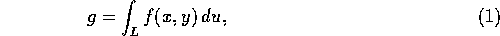where f(x,y) is the absorption coefficient of the object at the point (x,y) and L is the line along which the beam travels. Imaging systems such as CT scanners obtain g for various lines L, and use this information to compute an approximation to f(x,y) throughout the object.

Emission tomography, on the other hand, measures radiation emitted by an object of interest in various directions. In medical tomography, for example, a patient may be injected with a radioactive substance which enters the bloodstream. A movable array of detectors measures radiation arriving at each point from a direction perpendicular to the detector array (Fig. 1). Thus, at any given position of the detector array as it is rotated around the object, each detector measures radiation emitted by sources along a straight line. In the absence of attenuation, and with ideal detectors, the detected intensity will sum linearly along this line. If we denote this intensity g, then again:where f(x,y) represents the concentration of the radioactive substance at (x,y) and L represents the line passing through the detector perpendicular to the detector array.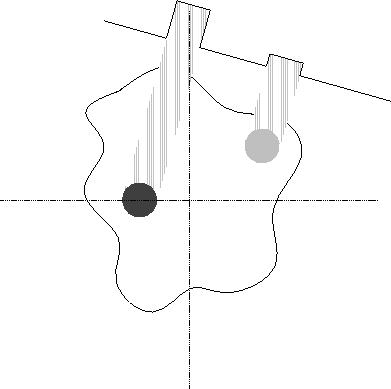Figure 1: Detection of a projection in emission tomography.

In each case, the mathematical formula for projection is the same. In the two-dimensional case, the line L can be represented uniquely by the parameters r and, wheremeasures the counterclockwise angle of the line from the vertical, and r measures the distance of the line from the origin of the (x,y) plane. Thus, we can use the above formula to define a transform which maps a function f(x,y) to a function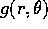, whereis the line integral of f(x,y) over the line defined by r and. This transform is known as the Radon transform and denoted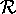. (Fig. 2). It corresponds closely to an ``ideal'' parallel-beam imaging system, since in such a system, each projection of the image corresponds to a slice through the radon transform at constant. The basic, idealized problem of tomography is to reconstruct an image from its Radon transform. Fortunately, the Radon transform, when properly defined, has a well-defined inverse . In order to invert the transform, one needs projection data spanning 180 degrees.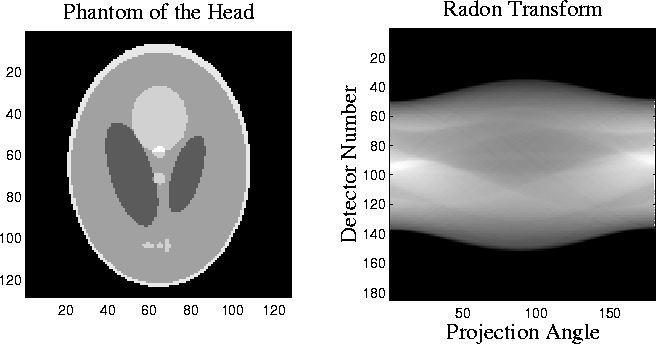Figure 2: The Shepp-Logan head phantom and its radon transform.is the counterclockwise angle from the horizontal to the line on which the detector array is located.

Unfortunately, the actual data detected by a medical imaging system does not correspond exactly to the Radon transform of the ``true'' image. In any imaging system, projection data will be corrupted by noise, and the projections are measured with only limited resolution. The geometry of the imaging system may differ from the ideal, particularly in transmission tomography, where a fan beam imaging system is more easily implemented than a parallel-beam system. Emission tomography suffers from the problem of attenuation. Since the object being imaged will itself absorb some of the emitted radiation, the observed intensity of a radioactive source depends on its distance from the detector and on the density of the substance between the detector and the source. In any type of tomography, the projection angles available may be limited. One may need to reconstruct an image from only a few projections, or from projections spanning only a small range of angles. In astronomical imaging, for example, one cannot necessarily obtain images of a heavenly body from all angles. Thus, the suitability of a reconstruction technique must be judged not only by its performance in the ideal case, but also by its potential to adapt to real imaging systems which differ from the ideal.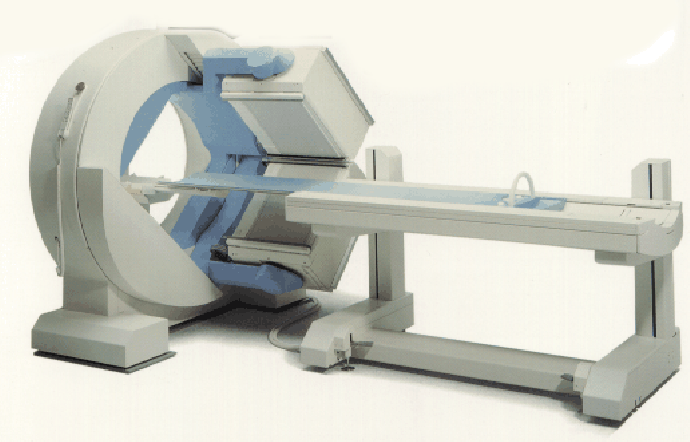Figure 3: Gamma camera (from Siemens).

In developing reconstruction techniques, we worked with both simulated data from ideal radon transforms, and with real data from a single-photon emission computed tomography (SPECT) system. The SPECT medical imaging system (Fig. 3) detects gamma rays emitted by a radioactive source. The camera head rotates around the subject. At each projection angle, gamma rays entering the camera head pass through an array of collimators which transmit only photons traveling in a direction perpendicular to the projection plane. The transmitted gamma rays then strike an array of fluorescent crystals. Photomultiplier tubes detect photons emitted by the crystals, localizing the location at which the original gamma ray struck the crystal sheet. Thus, this system produces projections with a parallel-beam geometry. From these projections, one can compute the distribution of radioactive emitters in the original image. The data which we used, provided by Dr. Richard Wendt of M.D. Anderson Cancer Center, was obtained by imaging a "phantom" similar to that shown in Fig. 4. The cylindrical plastic phantom is filled with radioactive (``hot'') water, into which are inserted vertical plastic rods which are not radioactive (``cold''). Thus, a horizontal slice of this phantom should be reconstructed as a bright circle with smaller dark circles in its interior corresponding to the lack of radioactivity within the cold rods (Fig. 4). The datafile with which we worked contained 240 projections of the phantom, obtained as the camera head moved in a full circle. Consecutive projection angles therefore differ by 1.5 degrees. Each projection is a 128 by 128 image. In order to work with the data, we extracted information corresponding to individual horizontal slices through the phantom from the original data file, which was in Interfile format. The reconstructed images in this paper represent the 80th slice through the phantom, a slice in which a number of rods should be visible.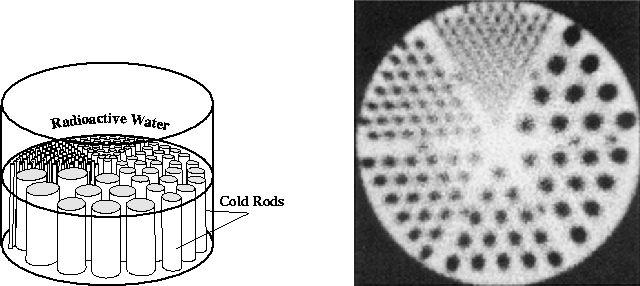Figure 4: SPECT phantom used in generating the data for this study (left), and the manufacturer's reconstruction of a slice through the phantom.

We approached the reconstruction problem using two different methods. The first, filtered back projection, is representative of the methods currently used in most commercial CT systems. It is fast and relatively effective, but difficult to adapt to imaging setups which differ from the ideal. The second method represents the wide class of algebraic reconstruction techniques (ART). These methods are more powerful than back projection in that they adapt easily to deviations in the imaging system from the ideal. Unfortunately, they are far more expensive to implement computationally, and are still more research topics than practical reconstruction techniques.Next: Filtered Back Projection Up: Tomographic Reconstruction of SPECT Previous: Tomographic Reconstruction of SPECT

Anders Johan Nygren
Thu May 8 12:28:25 CDT 1997